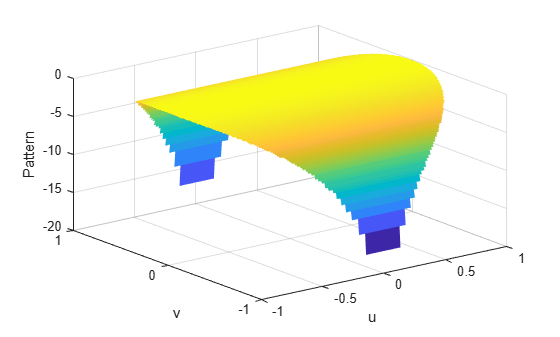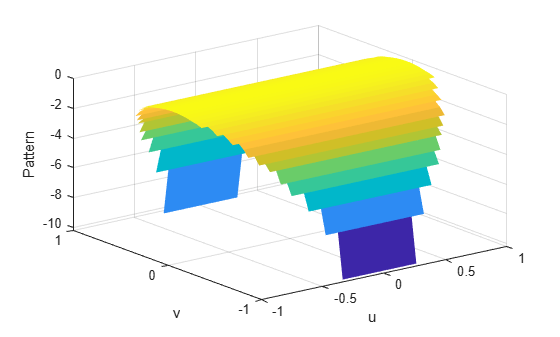# azel2uvpat

Convert radiation pattern from azimuth/elevation form to u/v form

## Syntax

``pat_uv = azel2uvpat(pat_azel,az,el)``
``pat_uv = azel2uvpat(pat_azel,az,el,u,v)``
``````[pat_uv,u_pat,v_pat] = azel2uvpat(___)``````

## Description

example

````pat_uv = azel2uvpat(pat_azel,az,el)` expresses the antenna radiation pattern `pat_azel` in u/v space coordinates instead of azimuth/elevation angle coordinates. `pat_azel` samples the pattern at azimuth angles in `az` and elevation angles in `el`. The `pat_uv` matrix uses a default grid that covers u values from –1 to 1 and v values from –1 to 1. In this grid, `pat_uv` is uniformly sampled with a step size of 0.01 for u and v. The function interpolates to estimate the response of the antenna at a given direction. Values in `pat_uv` are NaN for u and v values outside the unit circle because u and v are undefined outside the unit circle.```

example

````pat_uv = azel2uvpat(pat_azel,az,el,u,v)` uses vectors `u` and `v` to specify the grid at which to sample `pat_uv`. To avoid interpolation errors, `u` should cover the range [–1, 1] and `v` should cover the range [–1, 1].```

example

``````[pat_uv,u_pat,v_pat] = azel2uvpat(___)``` returns vectors containing the u and v coordinates at which `pat_uv` samples the pattern, using any of the input arguments in the previous syntaxes.```

## Examples

collapse all

Convert a radiation pattern to u-v space, with the u and v coordinates spaced by 0.01.

Define the pattern in terms of azimuth and elevation.

```az = -90:90; el = -90:90; pat_azel = mag2db(repmat(cosd(el)',1,numel(az)));```

Convert the pattern to u-v space.

`pat_uv = azel2uvpat(pat_azel,az,el);`

Plot the result of converting a radiation pattern to $u/v$ space with the $u$ and $v$ coordinates spaced by 0.01.

The radiation pattern is the cosine of the elevation angle.

```az = -90:90; el = -90:90; pat_azel = repmat(cosd(el)',1,numel(az));```

Convert the pattern to $u/v$ space. Use the $u$ and $v$ coordinates for plotting.

`[pat_uv,u,v] = azel2uvpat(pat_azel,az,el);`

Plot the result.

```H = surf(u,v,mag2db(pat_uv)); H.LineStyle = 'none'; xlabel('u'); ylabel('v'); zlabel('Pattern');```Convert a radiation pattern to $u/v$ form, with the $u$ and $v$ coordinates spaced by 0.05.

The radiation pattern is cosine of the elevation angle.

```az = -90:90; el = -90:90; pat_azel = repmat(cosd(el)',1,numel(az));```

Define the set of $u$ and $v$ coordinates at which to sample the pattern. Then, convert the pattern.

```u = -1:0.05:1; v = -1:0.05:1; pat_uv = azel2uvpat(pat_azel,az,el,u,v);```

Plot the result.

```H = surf(u,v,mag2db(pat_uv)); H.LineStyle = 'none'; xlabel('u'); ylabel('v'); zlabel('Pattern');```## Input Arguments

collapse all

Antenna radiation pattern in azimuth/elevation form, specified as a Q-by-P matrix. `pat_azel` samples the 3-D magnitude pattern in decibels, in terms of azimuth and elevation angles. P is the length of the `az` vector, and Q is the length of the `el` vector.

Data Types: `double`

Azimuth angles at which `pat_azel` samples the pattern, specified as a vector of length P. Each azimuth angle is in degrees, between –90 and 90. Such azimuth angles are in the hemisphere for which u and v are defined.

Data Types: `double`

Elevation angles at which `pat_azel` samples the pattern, specified as a vector of length Q. Each elevation angle is in degrees, between –90 and 90.

Data Types: `double`

u coordinates at which `pat_uv` samples the pattern, specified as a vector of length L. Each u coordinate is between –1 and 1.

Data Types: `double`

v coordinates at which `pat_uv` samples the pattern, specified as a vector of length M. Each v coordinate is between –1 and 1.

Data Types: `double`

## Output Arguments

collapse all

Antenna radiation pattern in u/v form, returned as an M-by-L matrix. `pat_uv` samples the 3-D magnitude pattern in decibels, in terms of u and v coordinates. L is the length of the `u` vector, and M is the length of the `v` vector. Values in `pat_uv` are NaN for u and v values outside the unit circle because u and v are undefined outside the unit circle.

u coordinates at which `pat_uv` samples the pattern, returned as a vector of length L.

v coordinates at which `pat_uv` samples the pattern, returned as a vector of length M.

collapse all

### Azimuth Angle, Elevation Angle

The azimuth angle of a vector is the angle between the x-axis and the orthogonal projection of the vector onto the xy plane. The angle is positive in going from the x axis toward the y axis. Azimuth angles lie between –180 and 180 degrees. The elevation angle is the angle between the vector and its orthogonal projection onto the xy-plane. The angle is positive when going toward the positive z-axis from the xy plane. By default, the boresight direction of an element or array is aligned with the positive x-axis. The boresight direction is the direction of the main lobe of an element or array.

Note

The elevation angle is sometimes defined in the literature as the angle a vector makes with the positive z-axis. The MATLAB® and Phased Array System Toolbox™ products do not use this definition.

This figure illustrates the azimuth angle and elevation angle for a vector shown as a green solid line.### U/V Space

The u and v coordinates are the direction cosines of a vector with respect to the y-axis and z-axis, respectively.

The u/v coordinates for the hemisphere x ≥ 0 are derived from the phi and theta angles by:

`$\begin{array}{l}u=\mathrm{sin}\theta \mathrm{cos}\varphi \\ v=\mathrm{sin}\theta \mathrm{sin}\varphi \end{array}$`

In these expressions, φ and θ are the phi and theta angles, respectively.

To convert azimuth and elevation to u and v use the transformation

`$\begin{array}{l}u=\mathrm{cos}el\mathrm{sin}az\\ v=\mathrm{sin}el\end{array}$`

which is valid only in the range abs(az)≤=90.

The values of u and v satisfy the inequalities

`$\begin{array}{l}-1\le u\le 1\\ -1\le v\le 1\\ {u}^{2}+{v}^{2}\le 1\end{array}$`

Conversely, the phi and theta angles can be written in terms of u and v using

`$\begin{array}{l}\mathrm{tan}\varphi =v/u\\ \mathrm{sin}\theta =\sqrt{{u}^{2}+{v}^{2}}\end{array}$`

The azimuth and elevation angles can also be written in terms of u and v:

`$\begin{array}{l}\mathrm{sin}el=v\\ \mathrm{tan}az=\frac{u}{\sqrt{1-{u}^{2}-{v}^{2}}}\end{array}$`

### Phi Angle, Theta Angle

The phi angle (φ) is the angle from the positive y-axis to the vector’s orthogonal projection onto the yz plane. The angle is positive toward the positive z-axis. The phi angle is between 0 and 360 degrees. The theta angle (θ) is the angle from the x-axis to the vector itself. The angle is positive toward the yz plane. The theta angle is between 0 and 180 degrees.

The figure illustrates phi and theta for a vector that appears as a green solid line.The coordinate transformations between φ/θ and az/el are described by the following equations

`$\begin{array}{l}\mathrm{sin}el=\mathrm{sin}\varphi \mathrm{sin}\theta \\ \mathrm{tan}az=\mathrm{cos}\varphi \mathrm{tan}\theta \\ \mathrm{cos}\theta =\mathrm{cos}el\mathrm{cos}az\\ \mathrm{tan}\varphi =\mathrm{tan}el/\mathrm{sin}az\end{array}$`

## Version History

Introduced in R2012a